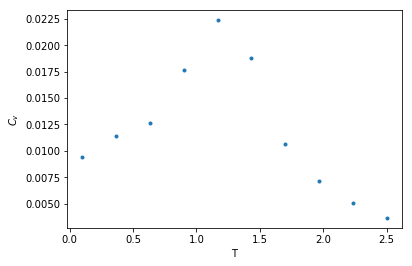# XY-MODEL

Monte Carlo simulation (Metropolis algorithm) on 2D XY-model

## How to use this program

#### import the class

``````from XY_model import XYSystem
``````

#### creating an object as a X-Y spin system with given width and temperature

Use `XYSystem(temperature = , width = )` to creat a class object. Two variables can be assigned to initilize the system: the temperature and the width.

``````xy_system_1 = XYSystem(temperature  = 0.5, width = 15)
``````

#### visulizingthe spin system

using `.show()` to visulize the xy spin system as arrows on two-dimensional plane.

``````xy_system_1.show()
print('Energy per spin:%.3f'%xy_system_1.energy)
``````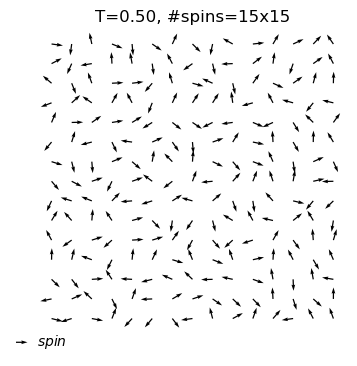``````Energy per spin:0.056
``````

#### Now, let the system evolve to a equilibrium state

`self.equilibrate(self,max_nsweeps=int(1e4),temperature=None,H=None,show = False)` allows you to assign a new temperature, just simply do object.equilibrate(temperature = 3). If you want to keep the temperature defined before, leave it blank. And ·sohw=Ture` will let show the configuration of the system for each 1000 sweeps.

``````xy_system_1.equilibrate(show=True)
xy_system_1.show()
``````
``````#sweeps=1
energy=-0.60
``````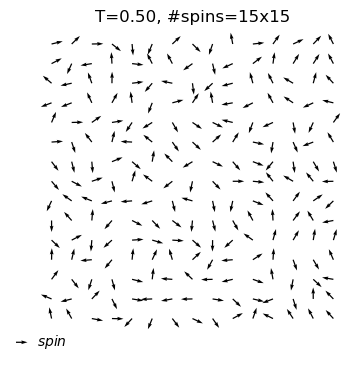``````equilibrium state is reached at T=0.5
#sweep=504
energy=-1.72
``````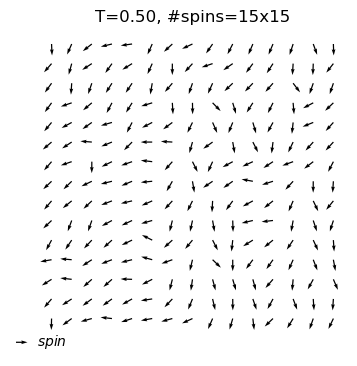#### Observing the thermal quantities in different temperature - annealing approach

``````xy_system_2 = XYSystem(width=7)
cool_dat=xy_system_2.annealing(T_init=2.5,T_final=0.1,nsteps = 10,show_equi=True)
``````
``````equilibrium state is reached at T=2.5
#sweep=6802
energy=-0.48
``````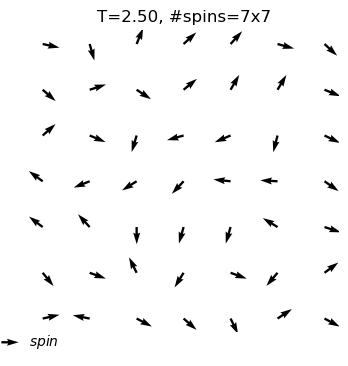``````equilibrium state is reached at T=2.2
#sweep=3429
energy=-0.48
``````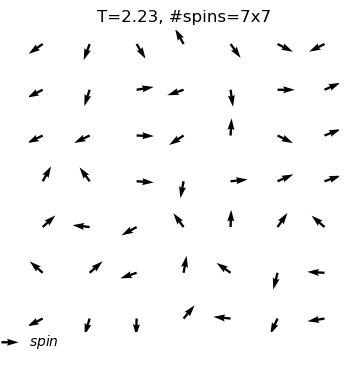``````equilibrium state is reached at T=2.0
#sweep=9999
energy=-0.47
``````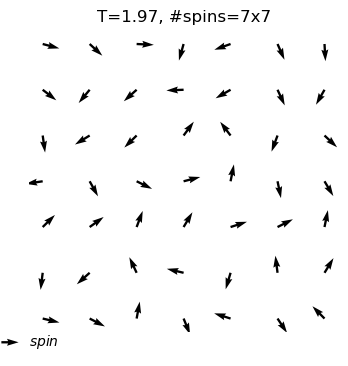``````equilibrium state is reached at T=1.7
#sweep=2809
energy=-0.60
``````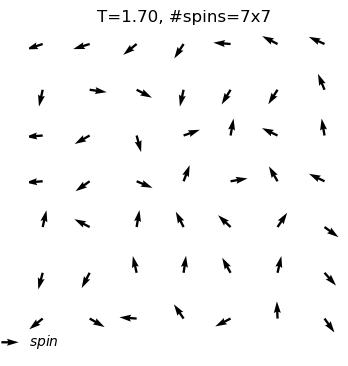``````equilibrium state is reached at T=1.4
#sweep=989
energy=-0.74
``````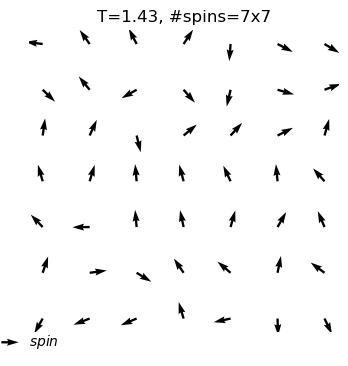``````equilibrium state is reached at T=1.2
#sweep=2253
energy=-1.35
``````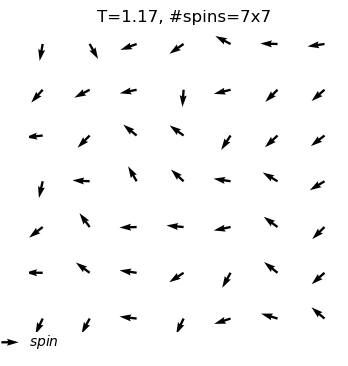``````equilibrium state is reached at T=0.9
#sweep=636
energy=-1.68
``````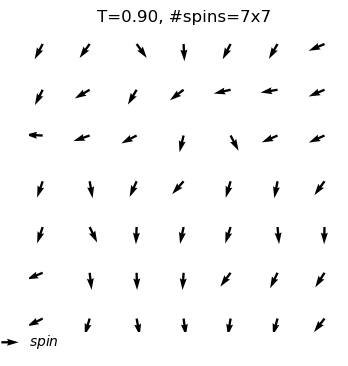``````equilibrium state is reached at T=0.6
#sweep=837
energy=-1.72
``````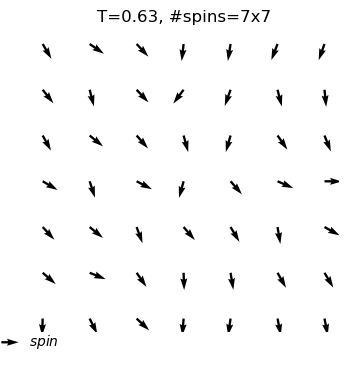``````equilibrium state is reached at T=0.4
#sweep=548
energy=-1.86
``````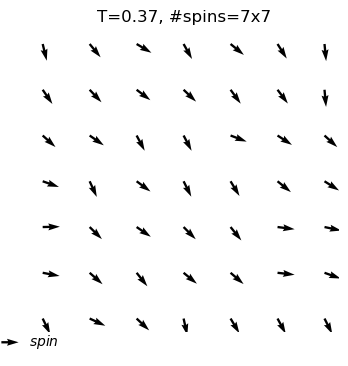``````equilibrium state is reached at T=0.1
#sweep=503
energy=-1.95
``````